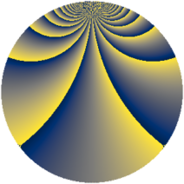# Properties

 Label 1216.4.beLevel $1216$ Weight $4$ Character orbit 1216.be Rep. character $\chi_{1216}(75,\cdot)$ Character field $\Q(\zeta_{16})$ Dimension $3824$ Sturm bound $640$

# Related objects

## Defining parameters

 Level: $$N$$ $$=$$ $$1216 = 2^{6} \cdot 19$$ Weight: $$k$$ $$=$$ $$4$$ Character orbit: $$[\chi]$$ $$=$$ 1216.be (of order $$16$$ and degree $$8$$) Character conductor: $$\operatorname{cond}(\chi)$$ $$=$$ $$1216$$ Character field: $$\Q(\zeta_{16})$$ Sturm bound: $$640$$

## Dimensions

The following table gives the dimensions of various subspaces of $$M_{4}(1216, [\chi])$$.

Total New Old
Modular forms 3856 3856 0
Cusp forms 3824 3824 0
Eisenstein series 32 32 0

## Trace form

 $$3824q - 16q^{4} - 16q^{5} - 16q^{6} - 16q^{7} - 16q^{9} + O(q^{10})$$ $$3824q - 16q^{4} - 16q^{5} - 16q^{6} - 16q^{7} - 16q^{9} - 16q^{11} - 16q^{16} - 16q^{17} - 8q^{19} - 16q^{20} - 16q^{23} - 16q^{24} - 16q^{25} - 96q^{26} + 1504q^{28} - 16q^{30} - 16q^{35} - 16q^{36} + 432q^{38} - 16q^{39} - 16q^{42} - 16q^{43} - 16q^{44} - 16q^{45} - 16q^{47} - 16q^{49} + 3440q^{54} - 16q^{55} - 8q^{57} - 16q^{58} - 16q^{61} + 3040q^{62} + 12080q^{64} + 10800q^{66} - 2080q^{68} - 16q^{73} - 16q^{74} + 5944q^{76} - 16q^{77} + 736q^{80} - 16q^{81} - 16q^{82} - 16q^{83} - 16q^{85} - 16q^{87} - 16q^{92} + 416q^{93} - 16q^{95} + 25824q^{96} - 448q^{99} + O(q^{100})$$

## Decomposition of $$S_{4}^{\mathrm{new}}(1216, [\chi])$$ into newform subspaces

The newforms in this space have not yet been added to the LMFDB.